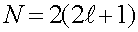Many-electron atoms

A second electron added to a hydrogen atom will see both the charge of the proton as well as the charge of the first electron. Altough a second electron can be slightly bound, one can obviously not add electrons indefinitely (In fact two is the most). Heavier nuclei, which have a larger number Z of protons can bind many electrons.

For our purposes, we will talk about energy levels of electrons in atoms without considering the effects of other electrons. The other electrons will for the most part rearrange the energy levels somewhat, but for purposes of classification and insight, it is good to classify the orbitals as if the other electrons were not there. The labels are the orbital and spin quantum numbers

For one electron in the vicinity of a single nucleus of charge Z, there are four quantum numbers which classify the electron, n, l, ml and ms. The first number n gives information about the energy of the orbital. Roughly, it describes the number of nodes in the wave function. The lowest energy level has n = 1, and the binding energy of the orbital falls inversely proportional to n2.The second numbers l and ml give information about the angular momentum of the state. Both are always integers. The angular momentum ml is in integral units of h/2p but never exceeds + l and never goes below -l. For instance if l equals 3, ml can take on the seven values, -3,2,-1,0,1,2,3. The values of l can be from zero to n - 1. There are therefore (2l + 1) values of ml for each l. The last quantum number ms is always +1/2 or -1/2, thus there are always two such numbers.

Due to the screening of other electrons the energies of various levels picks up a dependence on l as well as n. The levels are then denoted by n and l, and the two numbers ml and ms will be neglected except in knowing the number of different states that have a fixed n and l. That degeneracy isThe factor of two comes from the two values of ms and the other factor is for the different values of ml. Due to screenig, the lower values of l for a fixed n will have somewhat lower energy. For instance, if one considers an iron atom with 26 electrons, the electron shells for n < 5 that are:

 n l degeneracy 1 0 2 2 0 2 2 1 6 3 0 2 3 1 6 3 2 10 4 0 2 4 1 6 4 2 10 4 3 14

If an atom had a given number of electrons Ne, one would would fill the states from the top of the table down until all Ne electrons were exhausted.